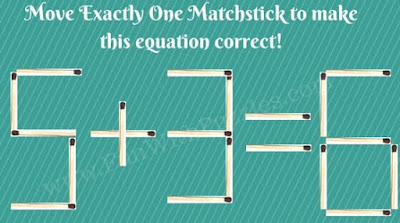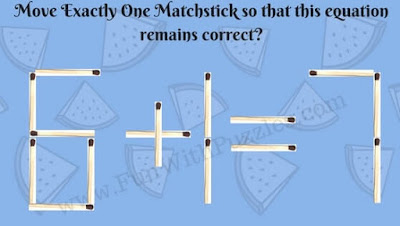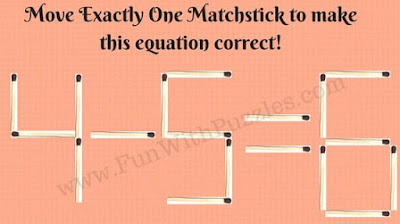There are many Mathematical Brain Teasers on this website. However, Matchstick Mathematical puzzles were missing until now. In the last few days, we have created many matchstick based puzzles which need to solved using mathematical skills. Today's matchstick puzzles are designed for kids and for the people who are first time doing these kinds of puzzles. In these matchstick picture puzzles, one has to move only one matchstick to solve the puzzle. Checkout Matchstick Picture Puzzles for teens in which one has to move two matchsticks to solve the puzzle. Also checkout Logical and Tricky Matchstick Picture Puzzles.
In these matchstick puzzles, you are presented with the mathematical equations with the matchsticks. Most of these math equations will not be correct. Your challenge is to move exactly one matchstick in each of these puzzles to make the equation correct. For some of the puzzles, there may be multiple ways to solve the equation.
Answers link to these matchstick puzzles is given at the end of this post. In case you can solve any of these puzzles in a different way, please do write your answer in the comment section of this post.1. Move exactly one matchstick to correct the equation2. Move exactly one matchstick to correct the equation3. Move exactly one matchstick to keep equation correct4. Move exactly one matchstick to correct the equation5. Move exactly one matchstick to correct the equation

Do checkout below mentioned Brain Teasers and Puzzles to increase your brain power

## List of Brain Enhancing Brain Teasers and Puzzles

1. Mathematical Logical Puzzles for Kids and Teens: These are brain teasers which are not only Mathematical but it will also test your logical reasoning.

2. Rapidly Maths Puzzles for Kids with Answers: These are easy Maths Brain Teasers for kids in which your challenge is to find the missing number which will replace the question mark.

3. Matchstick Puzzles for kids with answers: It contains another set of Matchstick Puzzles. However, these puzzles are not Mathematical puzzles but observational puzzles which will test your visual skills.

4. Will you crack the code? puzzles with answers: In these crack the code Picture Puzzles your task is to find the logical code which will open the lock.

Vicky said...

4-1=3
9-3=6
8-1=7
11-5=6
5+2=7